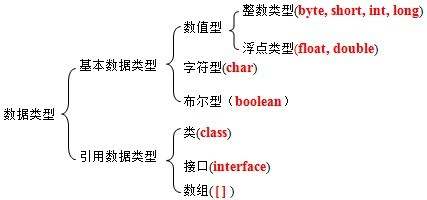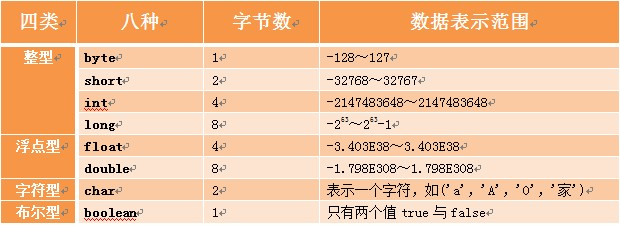# 数据类型简介

## Java数据分类

• 基本数据类型:描述一些具体的数字单元
• 数值型
• 整型:byte,short,int,long
• 浮点型:float,double
• 字符型:char
• 布尔型:boolean
• 引用数据类型:牵扯到内存关系的使用
• 类:class
• 接口:interface
• 数组:[]

• 如果需要描述数字首选int(整数),double(小数)
• 如果需要进行数据传输或是文字编码转换使用byte类型
• 处理中文使用char
• 描述内存或文件大小,表的主键列使用long

Java是一种强类型语言，针对每一种数据都给出了明确的数据类型，在内存中分配了不同大小的内存空间## 常量

### 常量分类

• 字符串常量:"HelloWorld"
• 整数常量:所有整数
• 小数常量:所有小数
• 字符常量:'A'
• 布尔常量:只有true和false
• 空常量:null

### 案例代码-常量

``````package 常量;
public class ChangLiang {
public static void main(String[] args) {
// 字符串常量
System.out.println("HelloWWorld");
// 整数常量
System.out.println(12);
System.out.println(-12);
// 小数常量
System.out.println(12.21);
// 字符常量
System.out.println('a');
System.out.println('0');
// 布尔常量
System.out.println(true);
System.out.println(false);
}
}``````

## 变量概述

### 变量定义格式

`数据类型 变量名 = 初始化值;`

### 案例代码-变量

``````package 变量;
public class BianLiang {
public static void main(String[] args) {
// 定义byte类型的变量
byte b = 10;
System.out.println(10);
System.out.println(b);

// 定义short类型的变量
short s = 100;
System.out.println(s);

// 定义int类型的变量
int i = 10000;
System.out.println(i);

// 定义long类型的变量
long l = 1000000000000000L;
System.out.println(l);

// 定义float类型的变量
float f = 12.34F;
System.out.println(f);

// 定义double类型的变量
double d = 12.34;
System.out.println(d);

// 定义char类型的变量
char c = 'a';
System.out.println(c);

// 定义boolean类型的变量
boolean bb = false;
System.out.println(bb);
}
}
``````
``````package 变量;
/*
变量定义注意事项：
1:变量未赋值,不能直接使用
2:变量只在它所属的范围内有效
变量属于它所在的那对大括号
3:一行上可以定义多个变量,但是不建议
*/
public class BianLiang2 {
public static void main(String[] args) {
// 定义变量
int a = 10;
System.out.println(a);
int b;
b = 20; // 变量在使用前赋值都是可以的
System.out.println(b);
{
int c = 100;
System.out.println(c);
}
// System.out.println(c); c定义在上面的括号内，只在上面括号内生效所以这里会报错
int aa = 10, bb = 20, cc = 30;
}
}
``````

# 整型数据类型

### 案例代码-定义int型变量

``````package Int;

public class IntDemo {
public static void main(String[] args) {
// int 变量名称 =常量
int x = 10; // 定义了一个整型变量x
// int型变量*int型变量=int型数据
x = 20;
System.out.println(x * x);
}
}``````

### 案例代码-超过范围

``````package Int;

public class IntDemo2 {
public static void main(String[] args) {
int max = Integer.MAX_VALUE; // 获取int最大值
int min = Integer.MIN_VALUE; // 获取int最小值
System.out.println(max);
System.out.println(min);
System.out.println("----------------");
// int型变量+int型常量=int型计算结果
System.out.println(max + 1); // -2147483648
System.out.println(min - 1); // 2147483647
}
}``````

# 浮点型数据类型

### 案例代码-定义double型变量

``````package Double;

public class DoubleDemo {
public static void main(String[] args) {
double x = 10.2;
int y = 10;
double result = x * y;
System.out.println(result);
}
}
``````

### 案例代码-定义float型变量

``````package Float;

public class FloatDemo {
public static void main(String[] args) {
float x = (float) 10.2;
float y = 10.1F;
System.out.println(x * y);
}
}
``````

# 字符型数据类型

### 案例代码-定义字符型变量

``````package Char;

public class CharDemo {
public static void main(String[] args) {
char a = 'a';
System.out.println(a);
}
}``````

``````package Char;

public class CharDemo2 {
public static void main(String[] args) {
char x = 'A';
int num = x;
System.out.println(num);
}
}
``````

# 布尔型数据类型

### 案例代码-定义布尔型数据

``````package Boolean;

public class BoolDemo {
public static void main(String[] args) {
boolean flag = true;
if (flag) {
System.out.println(true);
}
}
}``````

# String字符串

### 案例代码-定义布尔型数据

``````package String;

public class StringDermo {
public static void main(String[] args) {
String str = "Hello World!";
System.out.println(str);
}
}
``````

### 案例代码-字符串连接

``````package String;

public class StringDemo2 {
public static void main(String[] args) {
String str = "Hello";
String str2 = "Java";
System.out.println(str + str2);
}
}``````

``````package String;

public class StringDemo3 {
public static void main(String[] args) {
double x = 10.1;
int y = 2;
String str = "计算结果" + x + y;
System.out.println(str);
}
}
//计算结果10.12``````

Java中数据范围大的数据类型与数据范围小的数据类型进行计算时,所有数据范围小的转换喂数据大的数据类型,但是如果此时有String类型,则所有的类型无条件转换为String

``````package String;

public class StringDemo3 {
public static void main(String[] args) {
double x = 10.1;
int y = 2;
String str = "计算结果" + (x + y);
System.out.println(str);
}
}
``````

``````package String;

public class StringDemo4 {
public static void main(String[] args) {
System.out.println("\tHello \n \"World\"!!!");
}
}``````

=